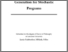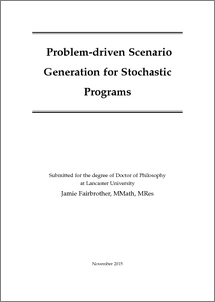# Problem-driven scenario generation for stochastic programs

Fairbrother, Jamie (2016) Problem-driven scenario generation for stochastic programs. PhD thesis, UNSPECIFIED.Preview
PDF (2016fairbrotherphd.pdf)
master.pdf - Published Version

## Abstract

Stochastic programming concerns mathematical programming in the presence of uncertainty. In a stochastic program uncertain parameters are modeled as random vectors and one aims to minimize the expectation, or some risk measure, of a loss function. However, stochastic programs are computationally intractable when the underlying uncertain parameters are modeled by continuous random vectors. Scenario generation is the construction of a finite discrete random vector to use within a stochastic program. Scenario generation can consist of the discretization of a parametric probabilistic model, or the direct construction of a discrete distribution. There is typically a trade-off here in the number of scenarios that are used: one must use enough to represent the uncertainty faithfully but not so many that the resultant problem is computationally intractable. Standard scenario generation methods are distribution-based, that is they do not take into account the underlying problem when constructing the discrete distribution. In this thesis we promote the idea of problem-based scenario generation. By taking into account the structure of the underlying problem one may be able to represent uncertainty in a more parsimonious way. The first two papers of this thesis focus on scenario generation for problems which use a tail-risk measure, such as the conditional value-at-risk, focusing in particular on portfolio selection problems. In the final paper we present a constraint driven approach to scenario generation for simple recourse problems, a class of stochastic programs for minimizing the expected shortfall and surplus of some resources with respect to uncertain demands.

Item Type:
Thesis (PhD)
ID Code:
82869
Deposited By:
Deposited On:
15 Nov 2016 09:40
Refereed?:
No
Published?:
Published